# Pears and oranges

There are 3 times as many pears as oranges. If a group of children receives 5 oranges each, there will be no oranges left over. If the same group of children receives 8 pears each, there will be 21 pears leftover. How many children and oranges are there?

c =  3
o =  15

### Step-by-step explanation:

p=3o
5c=o
8c+21=p

p=3•o
5•c=o
8•c+21=p

3o-p = 0
5c-o = 0
8c-p = -21

c = 3
o = 15
p = 45

Our linear equations calculator calculates it.
$o=15=15$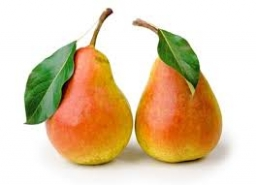Did you find an error or inaccuracy? Feel free to write us. Thank you!Tips to related online calculators
Do you have a system of equations and looking for calculator system of linear equations?

## Related math problems and questions:

• 1200 screws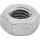1200 screws are to be divided into 3 groups so that in the 1st group there are 300 screws more than in the 2nd group and in the 2nd group 150 screws less than in the 3rd group. How many screws will be in each group?
• Oranges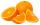Mother divided her three children's oranges in a ratio of 6:5:4. Two children gave 45 oranges. How many oranges were there?
• There 5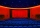There were 429 people at the play. Admission was 50 pesos each for adults and 35 pesos each for children. The receipts were 17265 pesos. How many adults and how many children attended?
• They boughtThey bought the students a set of notebooks for 65 euros. The first set cost € 2 and the second € 3. There were 5 times as many two-euro sets as three-set ones. How many sets of workbooks are there?
• Columns of two and threeWhen students in one class stand in columns of two, there is none left. When he stands in columns of three, there is one student left. There are 5 more double columns than three columns. How many students are in the class?In six baskets, the seller has fruit. In individual baskets, there are only apples or just pears with the following number of fruits: 5,6,12,14,23 and 29. "If I sell this basket," the salesman thinks, "then I will have just as many apples as a pear." Whic
• GroupA group of kids wanted to ride. When the children were divided into groups of 3 children, one remain. When divided into groups of 4 children, 1 remains. When divided into groups of 6 children, 1 remains. After divided into groups of 5 children, no one lef
• Group of childrenThere is a group of children. There is a boy named Adam in each of the three children subgroup and a girl named Beata in each quartet (four-member subgroup). How many children can be in such a group and what are their names in that case?
• Apples and pearsMom divided 24 apples and 15 pears to children. Each child received the same number of apples and pears - same number as his siblings. How many apples (j=?) and pears (h=?) received each child?
• At theAt the presentation of the travelers came three times as many men than women. When eight men left with their partners, there were five times more men than women at the presentation. How many were men and women originally?
• ChildrenThe group has 42 children. There are 4 more boys than girls. How many boys and girls are in the group?
• The sum 8The sum of two numbers is 21. If three times the smaller numbers is two less than twice the larger number, find the two numbers.
• Math logicThere are 20 children in the group, each two children have a different name. Alena and John are among them. How many ways can we choose 8 children to be among the selected A) was John B) was John and Alena C) at least one was Alena, John D) maximum one wa
• Candy and boxesWe have some candy and empty boxes. When we put candies in boxes of ten, there will be 2 candies and 8 empty boxes left. When of eight, there will be 6 candies and 3 boxes left. How many candy and empty boxes left when we put candies in boxes of nine?
• The cube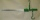The cube and cylinder weigh the same as three 20-gram weights. Three cubes and one cylinder weigh the same as two cylinders and one cube. How many grams does one cube weigh (and one cylinder)?
• PhotosThree friends Mirka, Lucka, and Hanka, collect photos. If Mirka had 3 more photos than she has, she would have as many as Hanka. If Lucka had 5 photos less than she has, she would be like Hanka. How many photographs does each have if they have 431 togethe
• Apples and pearsApples cost 50 cents piece, pears 60 cents piece, bananas cheaper than pears. Grandma bought 5 pieces of fruit. There was only one banana, and I paid 2 euros 75 cents. How many apples and how many pears?